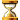### 31 – 32

Levels 31 – 32
Building
Level
Cost Cost Build(Accumulated)
Per Hour MaxWorkers
To reach
this level
(Accumulated)
Cost
per
player 
Cost
per
player
(Cumulative) 
Normal Normal + a
Forester's House 
Normal +
Helping
Hands

Normal +
Helping
Hands

and a
Forester's House 
Normal Normal +
Helping
Hands

31 1,995,717
(9,653,072)
 1,995,717 2= 997,859 3= 665,239 4= 498,929 5= 399,143 6= 332,620 7= 285,102 8= 249,465 9= 221,746 10= 199,572 11= 181,429 12= 166,310 13= 153,517 14= 142,551 15= 133,048 16= 124,732
 9,653,366 2= 4,826,683 3= 3,217,789 4= 2,413,342 5= 1,930,673 6= 1,608,894 7= 1,379,052 8= 1,206,671 9= 1,072,596 10= 965,337 11= 877,579 12= 804,447 13= 742,567 14= 689,526 15= 643,558 16= 603,335
 for 1= 1,996 2= 998 3= 666 4= 499 5= 400 6= 333 7= 286 8= 250 9= 222 10= 200 11= 182 12= 167 13= 154 14= 143 15= 134 16= 125
DISPLAYED:
1 D 12 h

1 D 12 h 23 m 19 s
(14 D 2 h 4 m 32 s)
758
 758 1 = 773 17 = 1,015 2 = 788 18 = 1,030 3 = 803 19 = 1,046 4 = 818 20 = 1,061 5 = 833 21 = 1,076 6 = 848 22 = 1,091 7 = 864 23 = 1,106 8 = 879 24 = 1,121 9 = 894 25 = 1,137 10 = 909 26 = 1,152 11 = 924 27 = 1,167 12 = 939 28 = 1,182 13 = 955 29 = 1,197 14 = 970 30 = 1,212 15 = 985 31 = 1,227 16 = 1,000 32 = 1,243
852
 852 1 = 869 17 = 1,142 2 = 886 18 = 1,159 3 = 903 19 = 1,176 4 = 920 20 = 1,193 5 = 938 21 = 1,210 6 = 955 22 = 1,227 7 = 972 23 = 1,245 8 = 989 24 = 1,262 9 = 1,006 25 = 1,279 10 = 1,023 26 = 1,296 11 = 1,040 27 = 1,313 12 = 1,057 28 = 1,330 13 = 1,074 29 = 1,347 14 = 1,091 30 = 1,364 15 = 1,108 31 = 1,381 16 = 1,125 32 = 1,398
758 1,137
32 2,311,042
(11,964,114)
 2,311,042 2= 1,155,521 3= 770,347 4= 577,761 5= 462,208 6= 385,174 7= 330,149 8= 288,880 9= 256,782 10= 231,104 11= 210,095 12= 192,587 13= 177,772 14= 165,074 15= 154,069 16= 144,440
 11,964,408 2= 5,982,204 3= 3,988,136 4= 2,991,102 5= 2,392,882 6= 1,994,068 7= 1,709,201 8= 1,495,551 9= 1,329,379 10= 1,196,441 11= 1,087,673 12= 997,034 13= 920,339 14= 854,601 15= 797,627 16= 747,776
 for 1= 2,312 2= 1,156 3= 771 4= 578 5= 463 6= 386 7= 331 8= 289 9= 257 10= 232 11= 211 12= 193 13= 178 14= 166 15= 155 16= 145
DISPLAYED:
1 D 16 h

1 D 16 h 13 m 39 s
(15 D 18 h 18 m 11 s)
#iferror:790
Building
Level
To reach
this level
(Accumulated)
Cost
per
player 
Cost
per
player
(Cumulative) 
Cost Build(Accumulated)
Normal Normal + a
Forester's House 
Normal +
Helping
Hands

Normal +
Helping
Hands

and a
Forester's House 
Normal Normal +
Helping
Hands

Cost Per Hour MaxWorkers

### Notes:

1. Based upon the cost for that mill level divided by the number of islanders.
2. Based on the cumulative cost of the saw mill level divided by the number of islanders.
3. With a building that increases production of the resource by 2% per each level of the building (max level=32 or a max increase of 64% in production).